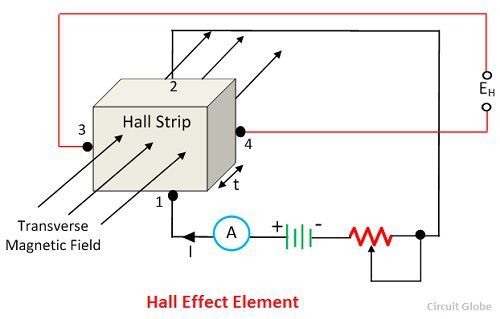# Hall Effect Transducer

Definition: The hall effect element is a type of transducer used for measuring the magnetic field by converting it into an emf. The direct measurement of the magnetic field is not possible. Thus the Hall Effect Transducer is used. The transducer converts the magnetic field into an electric quantity which is easily measured by the analogue and digital meters.

## Principle of Hall Effect Transducer

The principle of hall effect transducer is that if the current carrying strip of the conductor is placed in a transverse magnetic field, then the EMF develops on the edge of the conductor. The magnitude of the develop voltage depends on the density of flux, and this property of a conductor is called the Hall effect. The Hall effect element is mainly used for magnetic measurement and for sensing the current.

The metal and the semiconductor has the property of hall effect which depends on the densities and the mobility of the electrons.

Consider the hall effect element shown in the figure below. The current supply through the lead 1 and 2 and the output is obtained from the strip 3 and 4. The lead 3 and 4 are at same potential when no field is applied across the strip.When the magnetic field is applied to the strip, the output voltage develops across the output leads 3 and 4. The develops voltage is directly proportional to the strength of the material.

The I is the current in ampere and the B is the flux densities in Wb/m2

The current and magnetic field strength both can be measured with the help of the output voltages. The hall effect EMF is very small in conductors because of which it is difficult to measure. But semiconductors like germanium produces large EMF which is easily measured by the moving coil instrument.

### Applications of Hall Effect Transducer

The following are the application of the Hall effect Transducers.

1. Magnetic to Electric Transducer – The Hall effect element is used for converting the magnetic flux into an electric transducer. The magnetic fields are measured by placing the semiconductor material in the measurand magnetic field. The voltage develops at the end of the semiconductor strips, and this voltage is directly proportional to the magnetic field density.

The Hall Effect transducer requires small space and also gives the continuous signal concerning the magnetic field strength. The only disadvantage of the transducer is that it is highly sensitive to temperature and thus calibration requires in each case.

2. Measurement of Displacement – The Hall effect element measures the displacement of the structural element. For example – Consider the ferromagnetic structure which has a permanent magnet.The hall effect transducer placed between the poles of the permanent magnet. The magnetic field strength across the hall effect element changes by changing the position of the ferromagnetic field.

3. Measurement of Current – The hall effect transducer is also used for measuring the current without any physical connection between the conductor circuit and meter.

The AC or DC is applied across the conductor for developing the magnetic field. The strength of the magnetic field is directly proportional to the applied current. The magnetic field develops the emf across the strips. And this EMF depends on the strength of the conductor.

4. Measurement of Power  The hall effect transducer is used for measuring the power of the conductor. The current is applied across the conductor, which develops the magnetic field. The intensity of the field depends on the current. The magnetic field induces the voltage across the strip. The output voltage of the multiplier is proportional to the power of the transducer.